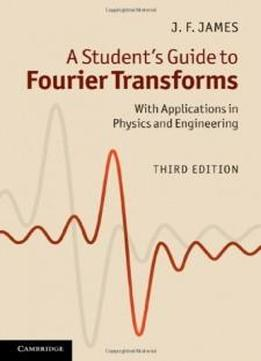# A Student's Guide To Fourier Transforms: With Applications In Physics And Engineering by J. F. James / 2011 / English / PDF

Fourier transform theory is of central importance in a vast range of applications in physical science, engineering and applied mathematics. Providing a concise introduction to the theory and practice of Fourier transforms, this book is invaluable to students of physics, electrical and electronic engineering, and computer science. After a brief description of the basic ideas and theorems, the power of the technique is illustrated through applications in optics, spectroscopy, electronics and telecommunications. The rarely discussed but important field of multi-dimensional Fourier theory is covered, including a description of Computer Axial Tomography (CAT scanning). The book concludes by discussing digital methods, with particular attention to the Fast Fourier Transform and its implementation. This new edition has been revised to include new and interesting material, such as convolution with a sinusoid, coherence, the Michelson stellar interferometer and the van Cittert-Zernike theorem, Babinet's principle and dipole arrays.

Fourier transform theory is of central importance in a vast range of applications in physical science, engineering and applied mathematics. Providing a concise introduction to the theory and practice of Fourier transforms, this book is invaluable to students of physics, electrical and electronic engineering, and computer science. After a brief description of the basic ideas and theorems, the power of the technique is illustrated through applications in optics, spectroscopy, electronics and telecommunications. The rarely discussed but important field of multi-dimensional Fourier theory is covered, including a description of Computer Axial Tomography (CAT scanning). The book concludes by discussing digital methods, with particular attention to the Fast Fourier Transform and its implementation. This new edition has been revised to include new and interesting material, such as convolution with a sinusoid, coherence, the Michelson stellar interferometer and the van Cittert-Zernike theorem, Babinet's principle and dipole arrays.

views: 650